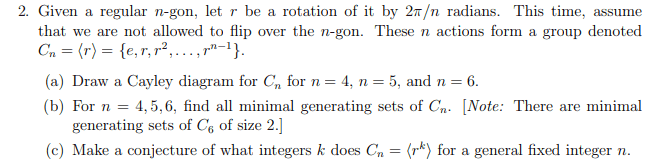# Group exercise for rotations of regular n-gon objects

LCSphysicist
Homework Statement:
\
Relevant Equations:
.The doubt is about B and C.

b)
n = 4, $C = {I,e^{2\pi/4}} n = 5,$C = {I,e^{2\pi/5}}
n = 6, $C = {I,e^{2\pi/6}} Is this right? c) I am not sure what does he wants... Last edited by a moderator: ## Answers and Replies Homework Helper Well, let's start by case ##n=4## in part b): What you have is essentially $$\langle e, r^2\rangle$$ But, from part a), if you did it correctly, you should know that ##r^4=e## and therefore $$\langle e, r^2\rangle = \{e, r^2\} \neq C_4$$ So no, what you have is not a generating set. The same is true for case ##n=6## where $$\langle e, r^2\rangle = \{e, r^2, r^4\}\neq C_6$$ You did it correctly for ##n=5## where $$\langle e, r^2\rangle = \{e, r^2, r^4, r, r^3\} = G_5$$ Now, you should first correct the cases ##n=4,6## and then, for case ##n=5## is this the only minimal generating set? •Delta2 LCSphysicist Well, let's start by case ##n=4## in part b): What you have is essentially $$\langle e, r^2\rangle$$ But, from part a), if you did it correctly, you should know that ##r^4=e## and therefore $$\langle e, r^2\rangle = \{e, r^2\} \neq C_4$$ So no, what you have is not a generating set. The same is true for case ##n=6## where $$\langle e, r^2\rangle = \{e, r^2, r^4\}\neq C_6$$ You did it correctly for ##n=5## where $$\langle e, r^2\rangle = \{e, r^2, r^4, r, r^3\} = G_5$$ Now, you should first correct the cases ##n=4,6## and then, for case ##n=5## is this the only minimal generating set? Hello. I think i do not understand yet the b. You said that what i wrote in the first and third case is essentially the same as$\langle e, r^2\rangle$, but my aim was to be the same as$\langle e, r\rangle$. Can't i call it equal r? So technically$r^4 = e$Homework Helper Hello. I think i do not understand yet the b. You said that what i wrote in the first and third case is essentially the same as$\langle e, r^2\rangle$, but my aim was to be the same as$\langle e, r\rangle$. Can't i call it equal r? So technically$r^4 = e\$
Oh yes, sorry my mistake, I read the exponential of ##4\pi## instead of the ##2\pi##. Then yes what you wrote is just ##\langle e, r \rangle## and it works for ##C_4## and ##C_6##, but as I have shown you for ##C_5##, ##\langle e, r^2\rangle## is also a minimal generating set. So now the question is, are there more minimal generating sets? You need to find them all.